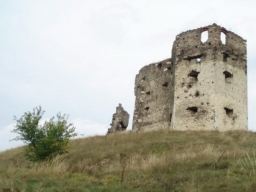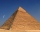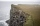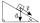# Depth angles

At the top of the mountain stands a castle, which has a tower 30 meters high. We see the crossroad in the valley from the top of the tower and heel at depth angles of 32° 50 'and 30° 10'. How high is the top of the mountain above the crossroad

Correct result:

y =  272.2651 m

#### Solution:We would be pleased if you find an error in the word problem, spelling mistakes, or inaccuracies and send it to us. Thank you!Tips to related online calculators

#### You need to know the following knowledge to solve this word math problem:

We encourage you to watch this tutorial video on this math problem:

## Next similar math problems:

• Trapezoid 25Trapezoid PART with AR||PT has (angle P=x) and (angle A=2x) . In addition, PA = AR = RT = s. Find the length of the median of Trapezoid PART in terms of s.
• GardensThe area of the square garden is 3/4 of the area of the triangular garden with sides of 80 m, 50 m, 50 m. How many meters of the fence do we need to fence a square garden?
• The staircaseThe staircase has a total height of 3.6 m and forms an angle of 26° with the horizontal. Calculate the length of the whole staircase.How long is a ladder that touches on a wall 4 meters high and its lower part is 3 meters away from the wall?
• Railway embankmentThe section of the railway embankment is an isosceles trapezoid, the sizes of the bases of which are in the ratio 5: 3. The arms have a length of 5 m and the height of the embankment is 4.8 m. Calculates the size of the embankment section area.
• The pyramidThe pyramid with a square base is 50 m high and the height of the sidewall is 80 m. Find the endge of the base of the pyramid.
• A cliffA line from the top of a cliff to the ground passes just over the top of a pole 5 ft high and meets the ground at a point 8 ft from the base of the pole. If the point is 93 ft from the base of the​ cliff, how high is the​ cliff?
• Isosceles triangleCalculate the area of an isosceles triangle, the base of which measures 16 cm and the arms 10 cm.
• Right triangleA right triangle ABC is given, c is a hypotenuse. Find the length of the sides a, b, the angle beta if c = 5 and angle alfa = A = 35 degrees.
• Isosceles triangleIn an isosceles triangle ABC with base AB; A [3,4]; B [1,6] and the vertex C lies on the line 5x - 6y - 16 = 0. Calculate the coordinates of vertex C.
• Chimney and treeCalculate the height of the factory chimney, which casts a shadow 6.5 m long in the afternoon. At the same time, a 6 m high tree standing near it casts a shadow 25 dm long.
• Find the 13Find the equation of the circle inscribed in the rhombus ABCD where A[1, -2], B[8, -3] and C[9, 4].
• Integer sidesA right triangle with an integer length of two sides has one leg √11 long. How much is its longest side?
• Powerplant chimneyFrom the window of the building at a height of 7.5 m, the top of the factory chimney can be seen at an altitude angle of 76° 30 ′. The base of the chimney can be seen from the same place at a depth angle of 5° 50 ′. How tall is the chimney?
• Trip with compassDuring the trip, Peter went 5 km straight north from the cottage, then 12 km west and finally returned straight to the cottage. How many kilometers did Peter cover during the whole trip?
• Inclined plane1. How much work W we have to do to pull a body weighing 200 kg along an inclined plane with a length of 4 m to a total height of 1.5 m. 2. Find the force we need to exert to do this if we neglect frictional resistance. 3. Find the force we would need if
• Right angleIn a right triangle ABC with a right angle at the apex C, we know the side length AB = 24 cm and the angle at the vertex B = 71°. Calculate the length of the legs of the triangle.